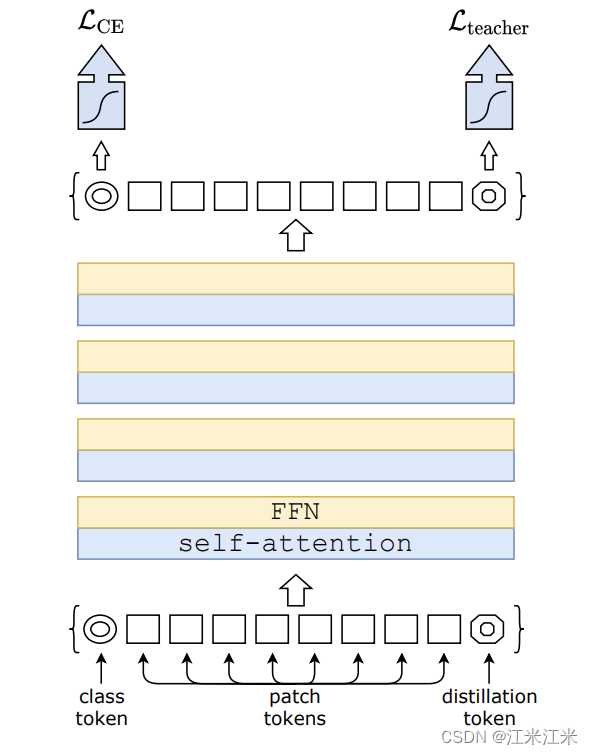+关注继续查看

# 概述

DEIT提出了一种基于token的蒸馏方法，使用和训练卷积网络差不多的时间，只用imagenet作为训练集，就实现了非常不错的效果。

• 证明了不包含卷积层的网络在只是用ImageNet数据的情况下也能取得很有竞争力的表现。
• 提出了一种基于token的蒸馏方法，并且这个方法的效果明显超过了普通的蒸馏方法。
• 有趣的是，基于transformer的模型以convnet为老师时表现的比以transformer为老师时要好。
• 他们的基于imagenet预训练的模型应用于其它下游任务时效果也很不错。

# Knowledge Distillation

T = params.temperature
KD_loss = nn.KLDivLoss()(F.log_softmax(outputs/T, dim=1),
F.softmax(teacher_outputs/T, dim=1)) * (alpha * T * T) + F.cross_entropy(outputs, labels) * (1. - alpha)


# DEIT

## base model: VIT

### transformer block

DEIT的工作是在VIT模型的基础上完成的。使用固定大小的RGB图像作为输入，这个图像被拆解成N个大小为16*16的小patch，N的大小一般是14*14。也就是说默认图像的大小是224*224。

self.proj = Rearrange('b c (h p) (w p ) -> b (h w) (p1 p2 c)', p = patch_size)
self.linear = nn.Linear(patch_size * patch_size * in_c, embed_dim)


self.proj = nn.Conv2d(in_c, embed_dim, kernel_size = patch_size, stride=patch_size)


### class token

VIT中模仿BERT的做法，在得到的patch embedding上concat了一个可训练的class token。这个class token也会贯穿整个网络，并且最终用于分类。它相当于起到了串联所有patch_embedding的作用，它包含的也是一个整体的信息。

### position embedding

self.qkv = nn.Linear(emb_size, emb_size*3)


 self.positions = nn.Parameter(torch.randn((img_size // patch_size) **2 + 1, emb_size))


We therefore perform 2D interpolation of the pre-trained position embeddings, according to their location in the original image.

## Distillation through attention

soft distillation

hard distillation

$$L^{hardDistill}_{global} = (1-\epsilon)\times\frac{1}{2}L_{CE}(\psi(Z_s),y) + \epsilon\times\frac{1}{2}L_{CE}(\psi(Z_s),yt)$$Distillation token

distillation token和class token一样，在整个训练过程中和别的token进行交互，并在最后一层输出。
class_token的分类结果向ground_truth靠齐，distillation_token的分类结果向我们的teacher靠齐。

# 代码实现

## DistilledVisionTransformer

class DistilledVisionTransformer(VisionTransformer):


### init()

self.dist_token = nn.Parameter(torch.zeros(1, 1, self.embed_dim))

self.cls_token = nn.Parameter(torch.zeros(1, 1, embed_dim)) if class_token else None


self.pos_embed = nn.Parameter(torch.zeros(1, num_patches + 2, self.embed_dim))


self.head_dist = nn.Linear(self.embed_dim, self.num_classes) if self.num_classes > 0 else nn.Identity()

 self.head = nn.Linear(self.embed_dim, num_classes) if num_classes > 0 else nn.Identity()


### forward()

1. 输入img，获得patch，并转成embedding的形式。
2. 增加cls embedding和position embedding。
3. 进入transformer encoder构成的blocks。每个block由两部分组成：
2. mlp
4. 进入mlp分类头，输出结果。

1. 输入img，获得patch，并转成embedding的形式。
2. 增加cls embedding和dist embedding和position embedding。
3. 进入transformer encoder构成的blocks。
4. cls token进入mlp分类头，dist token进入另一个分类头

#### embedding

if self.cls_token is not None:
x = torch.cat((self.cls_token.expand(x.shape, -1, -1), x), dim=1)
x = x + self.pos_embed


cls_tokens = self.cls_token.expand(B, -1, -1)  # stole cls_tokens impl from Phil Wang, thanks
dist_token = self.dist_token.expand(B, -1, -1)
x = torch.cat((cls_tokens, dist_token, x), dim=1)


#### logits

def forward_head(self, x, pre_logits: bool = False):
# 这里的x是self.forward_features的结果。
if self.global_pool:
x = x[:, self.num_prefix_tokens:].mean(dim=1) if self.global_pool == 'avg' else x[:, 0]
x = self.fc_norm(x)
return x if pre_logits else self.head(x)


 def forward(self, x):
x, x_dist = self.forward_features(x)
if self.training:
return x, x_dist
else:
# during inference, return the average of both classifier predictions
return (x + x_dist) / 2


## distillation loss

### init()

class DistillationLoss(torch.nn.Module):
"""
This module wraps a standard criterion and adds an extra knowledge distillation loss by
taking a teacher model prediction and using it as additional supervision.
"""
def __init__(self, base_criterion: torch.nn.Module, teacher_model: torch.nn.Module,
distillation_type: str, alpha: float, tau: float):
super().__init__()
self.base_criterion = base_criterion
self.teacher_model = teacher_model
assert distillation_type in ['none', 'soft', 'hard']
self.distillation_type = distillation_type
self.alpha = alpha
self.tau = tau


    if mixup_active:
# smoothing is handled with mixup label transform
criterion = SoftTargetCrossEntropy()
elif args.smoothing:
criterion = LabelSmoothingCrossEntropy(smoothing=args.smoothing)
else:
criterion = torch.nn.CrossEntropyLoss()

if args.bce_loss:
criterion = torch.nn.BCEWithLogitsLoss()


teacher_model.eval()


### forward()

def forward(self, inputs, outputs, labels)


base_loss = self.base_criterion(outputs, labels)


teacher_outputs = self.teacher_model(inputs)


T = self.tau
distillation_loss = F.kl_div(F.log_softmax(outputs_kd/T,dim=1), F.log_softmax(teacher_outputs/T, dim=1),reduction='sum',log_target=True)*(T*T)/outputs_kd.numel()
# We provide the teacher's targets in log probability because we use log_target=True


distillation_loss = F.cross_entropy(outputs_kd, teacher_outputs.argmax(dim=1))


loss = base_loss * (1 - self.alpha) + distillation_loss * self.alpha


|
2天前
|

工业视觉在生产和制造中扮演着关键角色，而缺陷检测则是确保产品质量和生产效率的重要环节。工业视觉的前景与发展在于其在生产制造领域的关键作用，尤其是在少样本缺陷检测方面，借助AidLux技术和深度学习分割模型UNET的实践应用，深度学习分割模型UNET的实践部署变得至关重要。
10 0
|
4月前
|

125 1
|
4月前
|

62 0
|
4月前
|

394 0
|
4月前
|

181 0
|
4月前
|

140 0
|
4月前
|

409 0
|
4月前
|

76 0
|
4月前
|

53 0
|
4月前
|

101 1